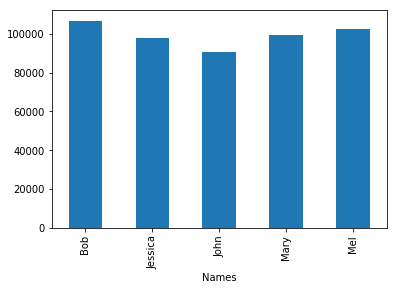# 学习Pandas，第 2 课

Numpy 将被用来生成一些样例数据。 我们开始课程的第一步是导入这些库。

``````# 导入所有需要的库
import pandas as pd
from numpy import random
import matplotlib.pyplot as plt
import sys #导入sys库只是为了确认一下Python的版本
import matplotlib #这样导入matplotlib只是为了显示一下其版本号

# 初始化matplotlib，用inline方式显示图形
%matplotlib inline
``````
``````print('Python version ' + sys.version)
print('Pandas version ' + pd.__version__)
print('Matplotlib version ' + matplotlib.__version__)
``````
``````Python version 3.6.1 | packaged by conda-forge | (default, Mar 23 2017, 21:57:00)
[GCC 4.2.1 Compatible Apple LLVM 6.1.0 (clang-602.0.53)]
Pandas version 0.19.2
Matplotlib version 2.0.2
``````

# 创建数据

``````# 婴儿名字的初始值
names = ['Bob','Jessica','Mary','John','Mel']
``````

• 生成一个 0 到 4 之间的随机数

``````# 这将确保随机样本是可以被重复生成的。
# 这也意味着生成的随机样本总是一样的。

random.seed?
``````
``````random.randint?
``````
``````len?
``````
``````range?
``````
``````zip?
``````

seed(500) - 创建一个种子

randint(low=0, high=len(names)) - 生成一个随机整数，介于 0 和 "names" 列表的长度之间。

names[n] - 从 "names" 列表中选择索引值(index)为 n 的名字。

for i in range(n) - 循环直到 i 等于 n，即: 1,2,3,....n。

random_names = 从 names 列表中选在一个随机名字并执行 n 次 (Select a random name from the name list and do this n times.)

``````random.seed(500)
random_names = [names[random.randint(low=0,high=len(names))] for i in range(1000)]

# 显示前10个名字
random_names[:10]
``````
``````['Mary',
'Jessica',
'Jessica',
'Bob',
'Jessica',
'Jessica',
'Jessica',
'Mary',
'Mary',
'Mary']
``````

``````# 1880年，不同婴儿名字对应的出生数量
births = [random.randint(low=0,high=1000) for i in range(1000)]
births[:10]
``````
``````[968, 155, 77, 578, 973, 124, 155, 403, 199, 191]
``````

zip 函数把 namesbirths 这两个数据集合并在一起。

``````BabyDataSet = list(zip(random_names,births))
BabyDataSet[:10]
``````
``````[('Mary', 968),
('Jessica', 155),
('Jessica', 77),
('Bob', 578),
('Jessica', 973),
('Jessica', 124),
('Jessica', 155),
('Mary', 403),
('Mary', 199),
('Mary', 191)]
``````

df 是一个 DataFrame对象。 你可以把这个对象理解为包含了 `BabyDataset` 的内容而格式非常象一个 sql 表格或者 Excel 的数据表。 让我们看看 df 中的内容。

``````df = pd.DataFrame(data = BabyDataSet, columns=['Names', 'Births'])
df[:10]
``````
Names Births
0 Mary 968
1 Jessica 155
2 Jessica 77
3 Bob 578
4 Jessica 973
5 Jessica 124
6 Jessica 155
7 Mary 403
8 Mary 199
9 Mary 191

``````df.to_csv?
``````

``````df.to_csv('births1880.txt', index=False, header=False)
``````

## 获取数据

``````pd.read_csv?
``````

``````Location = r'./births1880.txt'
``````

``````df.info()
``````
``````<class 'pandas.core.frame.DataFrame'>
RangeIndex: 999 entries, 0 to 998
Data columns (total 2 columns):
Mary    999 non-null object
968     999 non-null int64
dtypes: int64(1), object(1)
memory usage: 15.7+ KB
``````

• 数据集里有 999 条记录
• 有一列 Mary 有 999 个值
• 有一列 968 有 999 个值
• 列, 一个是numeric(数字型), 另外一个是non numeric(非数字型)

``````df.head()
``````
Mary 968
0 Jessica 155
1 Jessica 77
2 Bob 578
3 Jessica 973
4 Jessica 124

``````df = pd.read_csv(Location, header=None)
df.info()
``````
``````<class 'pandas.core.frame.DataFrame'>
RangeIndex: 1000 entries, 0 to 999
Data columns (total 2 columns):
0    1000 non-null object
1    1000 non-null int64
dtypes: int64(1), object(1)
memory usage: 15.7+ KB
``````

• 数据集里有 1000 条记录
• 有一列 0 有 1000 个值
• 有一列 1 有 1000 个值
• 列, 一个是numeric(数字型), 另外一个是non numeric(非数字型)

``````df.tail()
``````
0 1
995 John 151
996 Jessica 511
997 John 756
998 Jessica 294
999 John 152

``````df = pd.read_csv(Location, names=['Names','Births'])
``````
Names Births
0 Mary 968
1 Jessica 155
2 Jessica 77
3 Bob 578
4 Jessica 973

``````import os
os.remove(Location)
``````

## 准备数据

``````# 方法 1:
df['Names'].unique()
``````
``````array(['Mary', 'Jessica', 'Bob', 'John', 'Mel'], dtype=object)
``````
``````# 如果你想把这些值打印出来:
for x in df['Names'].unique():
print(x)
``````
``````Mary
Jessica
Bob
John
Mel
``````
``````# 方法 2:
print(df['Names'].describe())
``````
``````count     1000
unique       5
top        Bob
freq       206
Name: Names, dtype: object
``````

``````df.groupby?
``````
``````# 创建一个 groupby 的对象
name = df.groupby('Names')

# 在 groupby 对象上执行求和(sum)的功能
df = name.sum()
df
``````
Births
Names
Bob 106817
Jessica 97826
John 90705
Mary 99438
Mel 102319

## 分析数据

• 将 dataframe 排序并且找到第一行
• 使用 max() 属性找到最大值
``````# 方法 1:
Sorted = df.sort_values(['Births'], ascending=False)
``````
Births
Names
Bob 106817
``````# 方法 2:
df['Births'].max()
``````
``````106817
``````

## 表现数据

``````# Create graph
df['Births'].plot.bar()

print("The most popular name")
df.sort_values(by='Births', ascending=False)
``````
``````The most popular name
``````
Births
Names
Bob 106817
Mel 102319
Mary 99438
Jessica 97826
John 90705This tutorial was created by HEDARO

``````